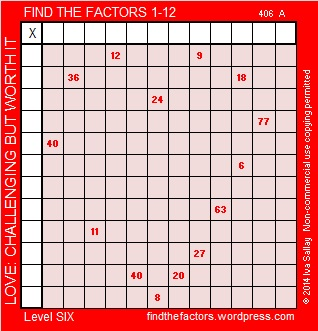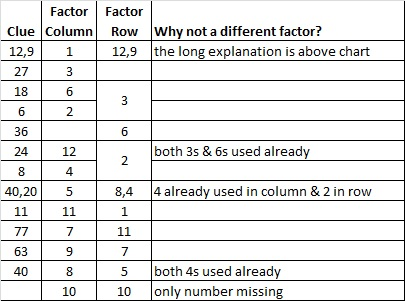# 62 and Love: Challenging But Worth It

62 is a composite number. 62 = 1 x 62 or 2 x 31. Factors of 62: 1, 2, 31, 62. Prime factorization: 62 = 2 x 31.62 is never a clue in the FIND THE FACTORS puzzles.All Previously Published PuzzlesHere is the logic to solve the puzzle & a chart showing one way to get the solution:

9 has to be the factor of both the 27 and the 63.  Therefore 3 x 6 = 18, (not 2 x 9).

3 is one of the factors of both the 18 and the 27.  Therefore 9 x 1 = 9, (not 3 x 3).This site uses Akismet to reduce spam. Learn how your comment data is processed.